MORE IN Circuits and Transmission Lines
MU Electronics and Telecom Engineering (Semester 3)
Circuits and Transmission Lines
May 2014
Total marks: --
Total time: --
INSTRUCTIONS
(1) Assume appropriate data and state your reasons
(2) Marks are given to the right of every question
(3) Draw neat diagrams wherever necessary

1 (a) Determine the z-parameter of the network shown in Fig 1(a) using y-z parameter relational only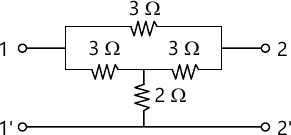5 M
1 (b) Test if F(s)=2s6+4s5+6s4+8s3+6s2+4s+2 is a Hurwitz polynomial
5 M
1 (c) Both the coils connected in series have self inductance of 40 mH. The total inductance of the circuit is found to be 40 mH. Determine the (i) mutual inductance between the coils and (ii) the coefficient of coupling
5 M
1 (d) Find Foster I and II and Cauer I and II circuits for the drving point admittance Y(s)=s+1
5 M

2 (a) Find the Thevenin equivalent across the terminal XY for the circuit shown in Fig. 2(a) using mesh matrix method. What should be the value of ? so that the circuit becomes reciprocal ?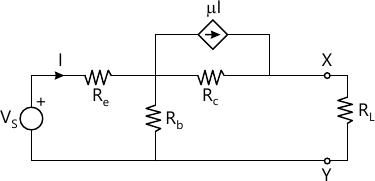10 M
2 (b) Find the magnitude of the controller source in the circuit shown in fig 2(b) by node analysis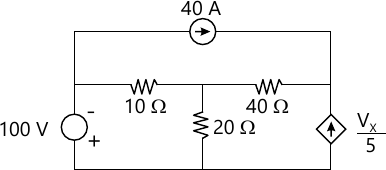5 M
2 (c) Check if the following polynomials are Hurwitz polynomials
(i) s+8 (ii) (s+8)7
5 M

3 (a) Synthesis the driving point function $F\left(s\right)=\frac{\left(s^2+4\right)\left(s^2+16\right)}{s\left(s^2+9\right)}$when F(s) is a driving point
(i) impedance (ii) admittance. Test if the circuit obtained are canonic
10 M
3 (b) Find the voltage transfer function of a loded two port network N in terms of the y-parameters (y11, y12, y21, y22) of the network N and load admittance YL
5 M
3 (c) The parameters of a transmission line are : G=2.25 m?/km, R=65 ?/km, L=1.6 mH/km, C=0.1 ?F/km. Find characteristics impedance and the propagation constant of the line at a frequency of 1 GHz
5 M

4 (a) Determine the z-parameters of the network shown in Fig. 3(a)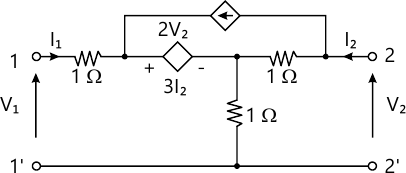10 M
4 (b) Determine the voltage transfer function for the circuit shown in Fig. 4(b) under the condition ?2L1C1=?2L2C2=1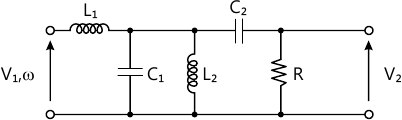5 M
4 (c) Test if $F\left(s\right)=\frac{s^3+6s^2+7s+7}{s^2+2s+1}$ is a Positive Real Function
5 M

5 (a) The network shown in Fig 5(a) attains steady-state with the switch K open. At t=0 the switch is closed. Determine the current through the switch at t=0+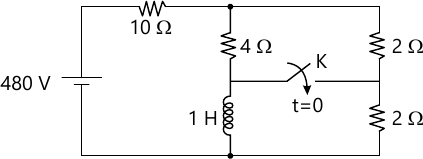10 M
5 (b) In the circuit shown in Fig 5(b) the two coupled coils have neglibible resistance. Find the current I2 when the input vi=10?2 sin 5000 tV.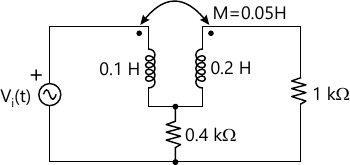5 M
5 (c) A generator of 1 V, 1 Khz supplies power to a 1000 km open write terminated in Zo having the following parameters.
R=10.4?/km
L=3.07mH/km
G=0.8 ?&Omgea;/km
C=0.00835 ?F/km
Calculate the power dilivered at the
5 M

6 (a) Find the expression for current i(t) through R in the circuit shown in Fig 6(a) using Laplace transform. Assume that the circuit is overdamped. Why is the frequency domain method prefered to the classical time domain method>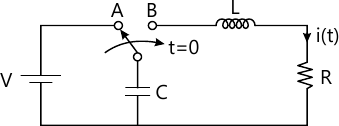10 M
6 (b) Find the voltage transfer function for the circuit shown in Fig.6(b). Assume L=CR2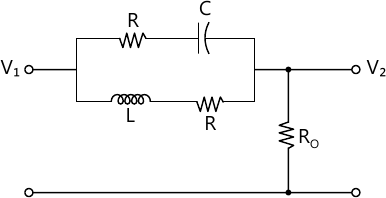5 M
6 (c) Draw the following normalized quantities on the Smith chart
(i) (2+j2) ?
(ii) (4-j2) ?
(iii) (1.0) ?
(iv) (j1.0) ?
5 M

More question papers from Circuits and Transmission Lines